#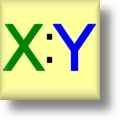Ratio Worksheets

## Ratio Worksheets for Teachers

Here is a graphic preview for all of the Ratio Worksheets. You can select different variables to customize these Ratio Worksheets for your needs. The Ratio Worksheets are randomly created and will never repeat so you have an endless supply of quality Ratio Worksheets to use in the classroom or at home. Our Ratio Worksheets are free to download, easy to use, and very flexible.

## Quick Link for All Ratio Worksheets

Click the image to be taken to that Ratio Worksheet.

#### SimpleRatio Worksheets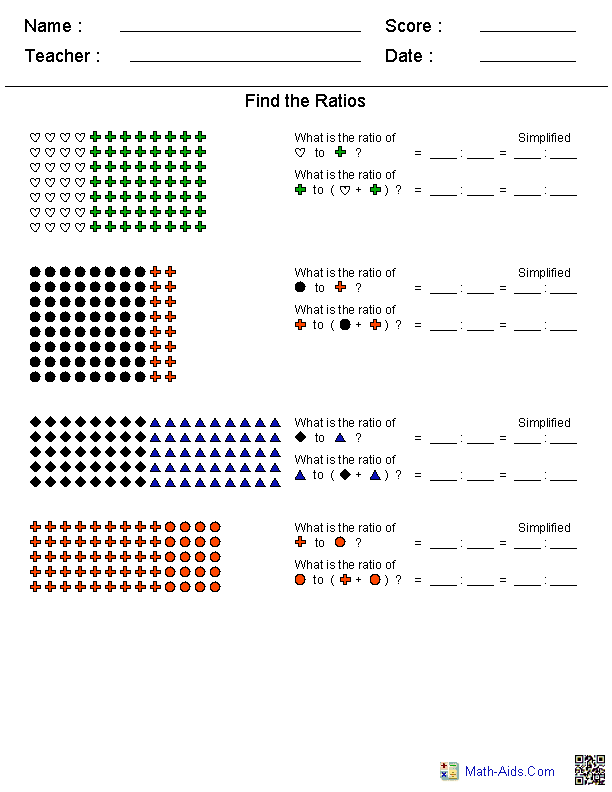#### EquivalentRatio Worksheets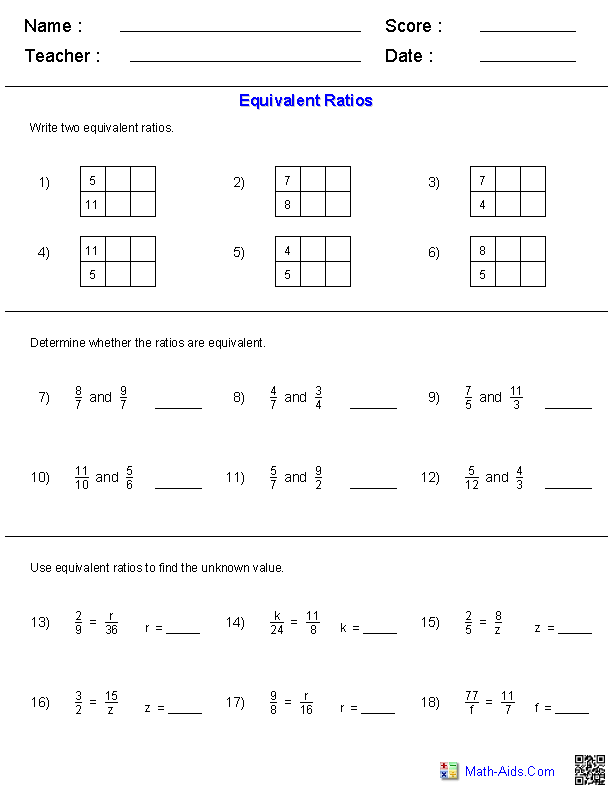#### Tables with EquivalentRatio Worksheets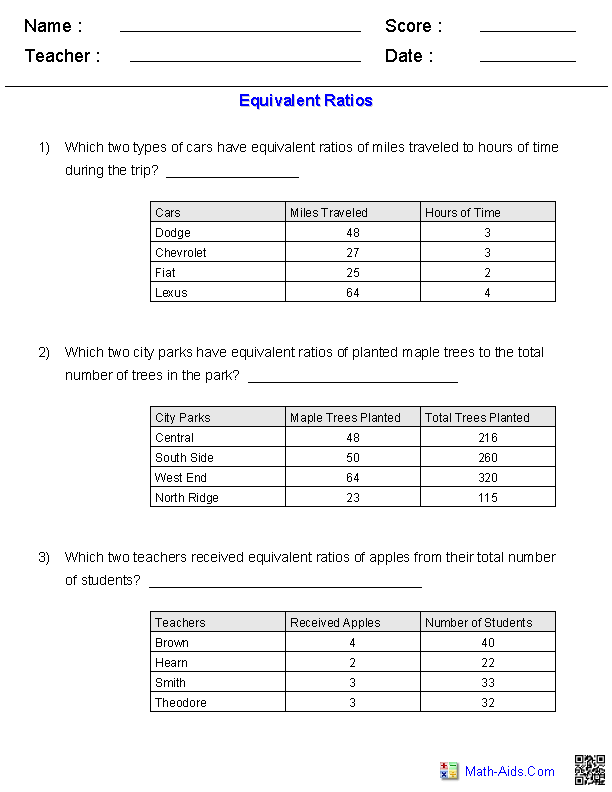#### Rows of EquivalentRatio Worksheets#### Ratios from Word PhrasesRatio Worksheets#### Rates and Unit RatesRatio Worksheets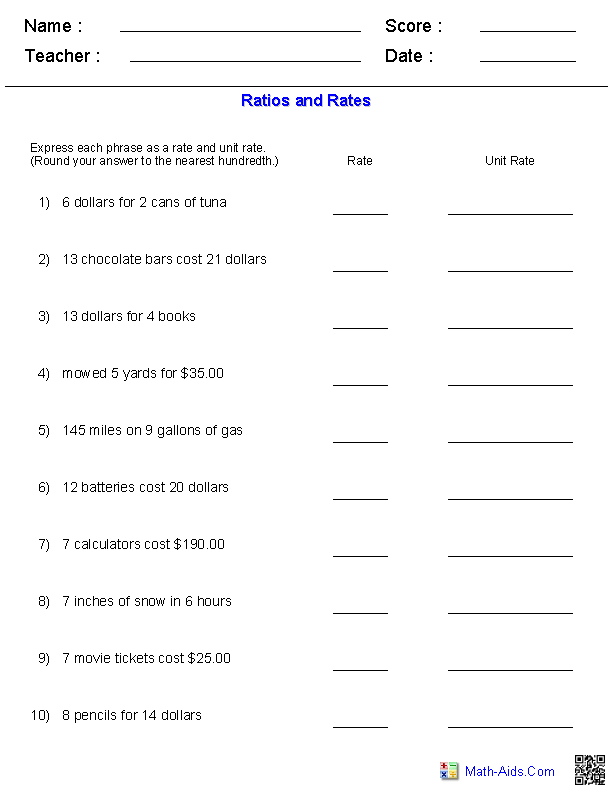#### Ratios and RatesRatio Worksheets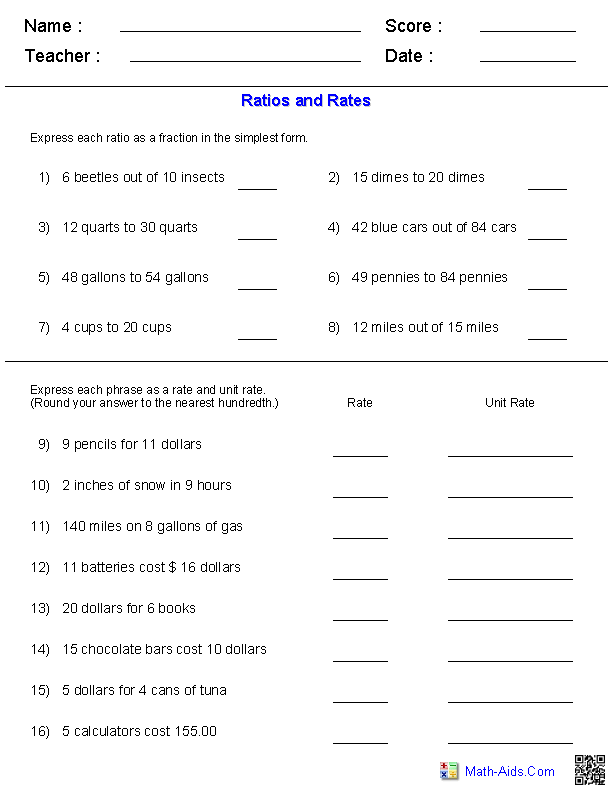#### Ratios and RateWord ProblemsRatio Worksheets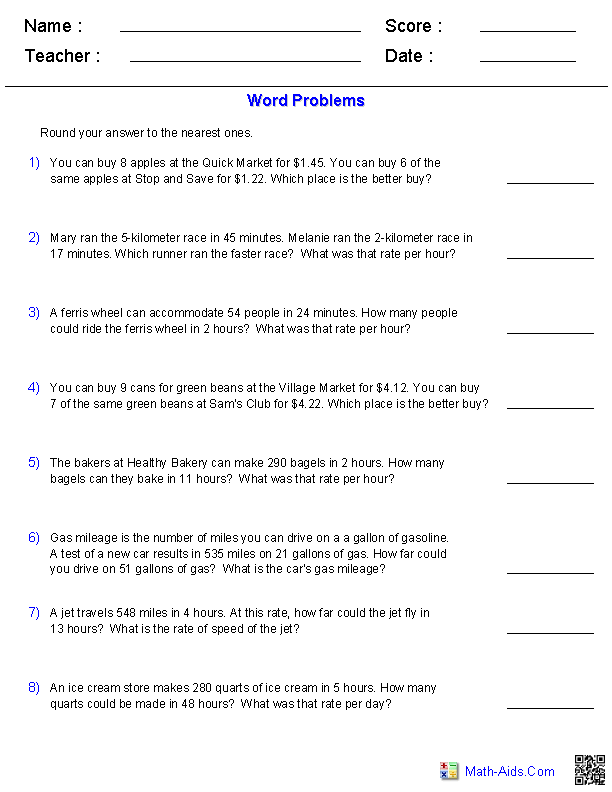Recommended Videos

## Detailed Description for All Ratio Worksheets

Simple Ratio Worksheets
These Ratio Worksheets will produce groups of symbols for the students to determine the ratio of the different symbols. These Ratio Worksheets are appropriate for 3rd Grade, 4th Grade, 5th Grade, 6th Grade, and 7th Grade.

Equivalent Ratio Worksheets
These Equivalent Ratio Worksheets will produce problems where the students must fill in a given table for a given ratio. They will be asked to identify if two ratios or equivalent and solve for unknown variables. These Ratio Worksheets are appropriate for 5th Grade, 6th Grade, and 7th Grade.

Tables with Equivalent Ratio Worksheets
These Equivalent Ratio Worksheets will will produce problems where the students must pick from a given table the two ratios that are equivalent. These Ratio Worksheets are appropriate for 5th Grade, 6th Grade, and 7th Grade.

Rows of Equivalent Ratio Worksheets
These Ratio Worksheets has rows of equivalent ratios, each with either the first or second term left blank. One ratio in the row of equivalent ratios will be written with both terms. The student will fill in the missing term for the equivalent ratio. These ratio worksheets will generate 10 Equivalent Ratio problems per worksheet. These Ratio Worksheets are appropriate for 3rd Grade, 4th Grade, 5th Grade, 6th Grade, and 7th Grade.

Ratios from Word Phrases Worksheets
These Ratio Worksheets will produce problems where the students must express the simplest form of a ratio from a word phrases. These ratio worksheets will generate 20 Ratio problems per worksheet. These Ratio Worksheets are appropriate for 3rd Grade, 4th Grade, 5th Grade, 6th Grade, and 7th Grade.

Rates and Unit Rates Worksheets
These Ratio Worksheets will produce problems where the students must write rates and unit rates from word phrases. These ratio worksheets will generate 10 Rates and Unit Rates problems per worksheet. These Ratio Worksheets are appropriate for 3rd Grade, 4th Grade, 5th Grade, 6th Grade, and 7th Grade.

Ratios and Rates Worksheets
These Ratio Worksheets will produce problems where the students must write simple fractions, rates, and unit rates from word phrases. These ratio worksheets will generate 16 Ratio and Rate problems per worksheet. These Ratio Worksheets are appropriate for 3rd Grade, 4th Grade, 5th Grade, 6th Grade, and 7th Grade.

Ratios and Rates Word Problems Worksheets
These Ratio Worksheets will produce eight ratio and rates word problems for the students to solve. These Ratio Worksheets are appropriate for 3rd Grade, 4th Grade, 5th Grade, 6th Grade, and 7th Grade.